# JP2018074761A - Control device for permanent magnet synchronous motor, control method, and image forming apparatus - Google Patents

Control device for permanent magnet synchronous motor, control method, and image forming apparatus Download PDF

## Info

Publication number
JP2018074761A
JP2018074761A JP2016212248A JP2016212248A JP2018074761A JP 2018074761 A JP2018074761 A JP 2018074761A JP 2016212248 A JP2016212248 A JP 2016212248A JP 2016212248 A JP2016212248 A JP 2016212248A JP 2018074761 A JP2018074761 A JP 2018074761A
Authority
JP
Japan
Prior art keywords
speed
estimated
unit
angle
permanent magnet
Prior art date
Legal status (The legal status is an assumption and is not a legal conclusion. Google has not performed a legal analysis and makes no representation as to the accuracy of the status listed.)
Pending
Application number
JP2016212248A
Other languages
Japanese (ja)
Inventor

Yasuhiro Koide

Yuta Tachibana

Daichi Suzuki

Hiroyuki Yoshikawa

Original Assignee
コニカミノルタ株式会社
Konica Minolta Inc
Priority date (The priority date is an assumption and is not a legal conclusion. Google has not performed a legal analysis and makes no representation as to the accuracy of the date listed.)
Filing date
Publication date
Application filed by コニカミノルタ株式会社, Konica Minolta Inc filed Critical コニカミノルタ株式会社
Priority to JP2016212248A priority Critical patent/JP2018074761A/en
Publication of JP2018074761A publication Critical patent/JP2018074761A/en
Pending legal-status Critical Current

• 230000001360 synchronised Effects 0.000 title claims abstract description 30
• 244000171263 Ribes grossularia Species 0.000 claims abstract description 105
• 230000000875 corresponding Effects 0.000 claims description 17
• 238000000034 methods Methods 0.000 claims description 15
• 230000001276 controlling effects Effects 0.000 claims description 3
• 230000001133 acceleration Effects 0.000 description 22
• 238000004804 winding Methods 0.000 description 13
• 239000004793 Polystyrene Substances 0.000 description 10
• 230000003247 decreasing Effects 0.000 description 8
• 230000004913 activation Effects 0.000 description 6
• 238000006243 chemical reactions Methods 0.000 description 5
• 238000003384 imaging method Methods 0.000 description 3
• 230000004048 modification Effects 0.000 description 3
• 238000006011 modification reactions Methods 0.000 description 3
• 230000003111 delayed Effects 0.000 description 2
• 230000000694 effects Effects 0.000 description 2
• 230000004907 flux Effects 0.000 description 2
• 238000010586 diagrams Methods 0.000 description 1
• 230000005284 excitation Effects 0.000 description 1
• 238000010438 heat treatment Methods 0.000 description 1
• 238000004519 manufacturing process Methods 0.000 description 1
• 238000003825 pressing Methods 0.000 description 1
• 230000004044 response Effects 0.000 description 1
• 230000001629 suppression Effects 0.000 description 1
• 230000001131 transforming Effects 0.000 description 1

## Images

•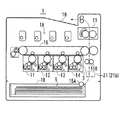•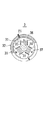•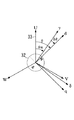•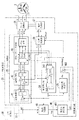•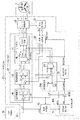•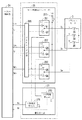•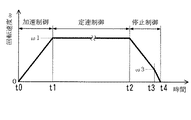•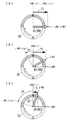•••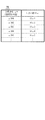•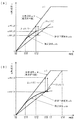•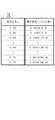•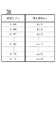••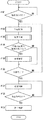•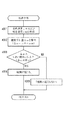••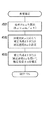## Classifications

• HELECTRICITY
• H02GENERATION; CONVERSION OR DISTRIBUTION OF ELECTRIC POWER
• H02PCONTROL OR REGULATION OF ELECTRIC MOTORS, ELECTRIC GENERATORS OR DYNAMO-ELECTRIC CONVERTERS; CONTROLLING TRANSFORMERS, REACTORS OR CHOKE COILS
• H02P21/00Arrangements or methods for the control of electric machines by vector control, e.g. by control of field orientation
• H02P21/06Rotor flux based control involving the use of rotor position or rotor speed sensors
• HELECTRICITY
• H02GENERATION; CONVERSION OR DISTRIBUTION OF ELECTRIC POWER
• H02PCONTROL OR REGULATION OF ELECTRIC MOTORS, ELECTRIC GENERATORS OR DYNAMO-ELECTRIC CONVERTERS; CONTROLLING TRANSFORMERS, REACTORS OR CHOKE COILS
• H02P21/00Arrangements or methods for the control of electric machines by vector control, e.g. by control of field orientation
• H02P21/14Estimation or adaptation of machine parameters, e.g. flux, current or voltage
• H02P21/18Estimation of position or speed
• HELECTRICITY
• H02GENERATION; CONVERSION OR DISTRIBUTION OF ELECTRIC POWER
• H02PCONTROL OR REGULATION OF ELECTRIC MOTORS, ELECTRIC GENERATORS OR DYNAMO-ELECTRIC CONVERTERS; CONTROLLING TRANSFORMERS, REACTORS OR CHOKE COILS
• H02P21/00Arrangements or methods for the control of electric machines by vector control, e.g. by control of field orientation
• H02P21/24Vector control not involving the use of rotor position or rotor speed sensors
• H02P21/26Rotor flux based control
• HELECTRICITY
• H02GENERATION; CONVERSION OR DISTRIBUTION OF ELECTRIC POWER
• H02PCONTROL OR REGULATION OF ELECTRIC MOTORS, ELECTRIC GENERATORS OR DYNAMO-ELECTRIC CONVERTERS; CONTROLLING TRANSFORMERS, REACTORS OR CHOKE COILS
• H02P25/00Arrangements or methods for the control of AC motors characterised by the kind of AC motor or by structural details
• H02P25/02Arrangements or methods for the control of AC motors characterised by the kind of AC motor or by structural details characterised by the kind of motor
• H02P25/022Synchronous motors
• H02P25/03Synchronous motors with brushless excitation
• HELECTRICITY
• H02GENERATION; CONVERSION OR DISTRIBUTION OF ELECTRIC POWER
• H02PCONTROL OR REGULATION OF ELECTRIC MOTORS, ELECTRIC GENERATORS OR DYNAMO-ELECTRIC CONVERTERS; CONTROLLING TRANSFORMERS, REACTORS OR CHOKE COILS
• H02P6/00Arrangements for controlling synchronous motors or other dynamo-electric motors using electronic commutation dependent on the rotor position; Electronic commutators therefor
• H02P6/14Electronic commutators
• H02P6/16Circuit arrangements for detecting position
• H02P6/18Circuit arrangements for detecting position without separate position detecting elements
• HELECTRICITY
• H02GENERATION; CONVERSION OR DISTRIBUTION OF ELECTRIC POWER
• H02PCONTROL OR REGULATION OF ELECTRIC MOTORS, ELECTRIC GENERATORS OR DYNAMO-ELECTRIC CONVERTERS; CONTROLLING TRANSFORMERS, REACTORS OR CHOKE COILS
• H02P2207/00Indexing scheme relating to controlling arrangements characterised by the type of motor
• H02P2207/05Synchronous machines, e.g. with permanent magnets or DC excitation

## Abstract

PROBLEM TO BE SOLVED: To prevent out-of-synchronism caused by displacement between an actual position of a magnetic pole and an estimated position.SOLUTION: A control device 21 for a permanent magnet synchronous motor 3 comprises: a drive part 26 which drives a rotor by causing a current to flow to an armature 31; a speed estimation part which estimates a rotation speed of the rotor based on the current flowing to the armature 31; a magnetic pole position estimation part 24 which estimates a magnetic pole position of the rotor based on an estimated speed that is the estimated rotation speed; a control part 23 for controlling the drive part 26 so as to generate a rotary magnetic field that is rotated at a target speed ω* indicated by an inputted speed command S1 based on an estimated angle θm that is an estimate of the magnetic pole position outputted from the magnetic pole position estimation part 24; an out-of-synchronism prediction part 45 which predicts whether out-of-synchronism occurs based on the target speed ω* and the estimated angle θm; and a correction part 46 which corrects the estimated angle θm in a case where the out-of-synchronism prediction part 45 predicts that out-of-synchronism may occur.SELECTED DRAWING: Figure 4

## Description

The present invention relates to a control device, a control method, and an image forming apparatus for a permanent magnet synchronous motor.

In general, a permanent magnet synchronous motor (PMSM) has a stator having a winding and a rotor using a permanent magnet, and a rotating magnetic field is generated by flowing an alternating current through the winding to generate a rotating magnetic field. Rotate the child synchronously with it. According to the vector control that controls the alternating current as a vector component in the dq coordinate system, it can be efficiently and smoothly rotated.

In recent years, sensorless permanent magnet synchronous motors have been widely used. The sensorless type does not have a magnetic sensor or an encoder for detecting the magnetic pole position. For this reason, in the vector control of the sensorless permanent magnet synchronous motor, the rotational speed (angular speed) of the rotor and the magnetic pole are determined by a predetermined arithmetic expression such as an expression of the dq axis model based on the current or voltage flowing through the winding. A method for estimating the position (angle) is used. In general, the magnetic pole position is estimated by an integral operation based on the rotation speed.

The estimated value (estimated speed) of the rotational speed is used for setting a current command value that determines the magnitude of the current flowing through the shoreline. That is, a setting is generally made such that the current command value is increased when the estimated speed is smaller than the target speed and the current command value is decreased when the estimated speed becomes larger than the target speed so that the estimated speed approaches the target speed. With regard to such a setting, for example, in Patent Document 1, in a driving state in which the d-axis current in the magnetic flux direction is zero, a q-axis that generates torque for rotational driving when the estimated speed deviates from a predetermined speed limit range. It describes that the current command value of the current is increased or decreased.

The estimated value (estimated angle) of the magnetic pole position is fed back to vector control with a coordinate conversion calculation for generating a control signal to be given to the permanent magnet synchronous motor based on the set current command value and a measured value of the current flowing through the winding. It is generally used for coordinate transformation calculations for the purpose.

As a prior art for improving the accuracy of estimation of the magnetic pole position, there is a technique described in Patent Document 2. In Patent Document 2, an actual value of a rotational speed is calculated based on a position detection signal Sa input from an actuator driven by a motor, and an estimated angle is corrected based on a difference between the actual value and an estimated speed. Is described.

JP2012-062909A Japanese Patent Laying-Open No. 2015-133872

Conventionally, in the control of a permanent magnet synchronous motor, a step-out may occur, and there is a problem that the step-out is likely to occur particularly at the time of starting to accelerate the rotation.

When the rotation is accelerating, the rotation speed increases from the estimation of the rotation speed until the estimated value is reflected in the drive. That is, the estimation of the rotation speed is delayed with respect to the change in the rotation speed. The estimation delay of the rotational speed is integrated in the estimation of the magnetic pole position based on the estimated speed. For this reason, a large delay is likely to occur in the estimated angle that is the estimated value of the magnetic pole position.

At startup, if the estimated angle delay increases, the torque decreases with respect to the actual magnetic pole position of the rotor. For this reason, there is a high possibility that the rotation speed decreases and step-out occurs.

As a method of compensating for the decrease in torque, there is a method of increasing the current flowing through the winding. However, when the magnitude of the current is set to the upper limit of the settable range determined by the specifications of the drive circuit, the current cannot be increased further. Generally, at the time of start-up, the current is set near the upper limit of the settable range, so the current cannot be increased further. If the upper limit of the settable range is increased by changing the components of the drive circuit, the manufacturing cost of the drive circuit will increase.

The technique described in Patent Document 2 described above is to correct the estimated angle by acquiring a position detection signal Sa corresponding to the angular position of the rotor from a sensor provided outside the permanent magnet synchronous motor. That is, even if the permanent magnet synchronous motor is a sensorless type, the control device for the permanent magnet synchronous motor has a sensor for detecting the position of the rotor, and the cost reduction effect of using the sensorless type permanent magnet synchronous motor is achieved. Damaged.

As another factor causing the step-out, a difference between a plurality of parameter values (motor constants) used for calculation for estimating the rotation speed and the magnetic pole position and the actual parameter value can be considered. The parameters include the resistance value of the winding, the inductance of the winding, and the induced voltage constant. When the temperature of the winding or the permanent magnet changes due to the drive of the permanent magnet synchronous motor or the change of the ambient temperature, a deviation occurs between the parameter value set in advance for estimation and the actual parameter value. Therefore, the magnetic pole position and the rotation speed may not be correctly estimated.

Regarding the deviation of the parameter value, for example, there is a method of updating the parameter value used for the calculation by measuring each parameter value at each activation. However, in this case, the time required for each activation becomes longer, and the response to the activation command is reduced.

The present invention has been made in view of the above-described problems, and an object thereof is to provide a control device and a control method that can prevent a step-out caused by a deviation between an actual position of a magnetic pole and an estimated position. .

A control device according to an embodiment of the present invention is a control device for a permanent magnet synchronous motor in which a rotor using a permanent magnet is rotated by a rotating magnetic field due to a current flowing in the armature, and the current is passed through the armature to A driving unit that drives the rotor, a speed estimation unit that estimates a rotational speed of the rotor based on a current flowing through the armature, and a magnetic pole of the rotor based on an estimated speed that is the estimated rotational speed A magnetic field position estimation unit that estimates a position, and the rotating magnetic field that rotates at a target speed indicated by an input speed command is generated based on an estimated angle that is an estimated value of the magnetic pole position output from the magnetic pole position estimation unit. When the step-out occurs by the control unit that controls the drive unit, the step-out prediction unit that predicts whether or not step-out occurs based on the target speed and the estimated speed, and the step-out prediction unit prediction A correction unit that corrects the estimated angle when the estimated angle is corrected, and the control unit corrects the estimated angle that is corrected when the estimated angle is corrected by the correction unit. Based on the above, the drive unit is controlled so that the rotating magnetic field corresponding to the target speed is generated.

ADVANTAGE OF THE INVENTION According to this invention, the control apparatus and control method which can prevent the step-out resulting from the shift | offset | difference of the actual position of a magnetic pole and the estimated position can be provided.

1 is a diagram illustrating an outline of a configuration of an image forming apparatus including a motor control device according to an embodiment of the present invention. It is a figure which shows typically the structure of a brushless motor. It is a figure which shows the dq axis | shaft model of a brushless motor. It is a figure which shows an example of a functional structure of a motor control apparatus. It is a figure which shows the modification of the functional structure of a motor control apparatus. It is a figure which shows the example of a structure of a motor drive part and an electric current detection part. It is a figure which shows the example of the driving | running pattern of a brushless motor. It is a figure which shows the relationship between the shift | offset | difference of an estimated angle, and a drive torque. It is a figure which shows the example of the setting of the threshold value used for prediction of a step-out. It is a figure which shows the example of the setting of the upper limit of the delay amount of an estimated angle. It is a figure which shows the example of the table which shows the threshold value according to target speed. It is a figure which shows the example of transition of an estimated angle. It is a figure which shows the example of the table which shows the correction | amendment angle according to speed ratio. It is a figure which shows the example of the table which shows the correction speed according to speed ratio. It is a figure which shows the other example of transition of an estimated angle. It is a figure which shows the flow of a process in a motor control apparatus. It is a figure which shows the example of the flow of a process of step-out prediction. It is a figure which shows the example of the flow of a process of step-out prediction. It is a figure which shows the example of the flow of a process of angle correction | amendment.

FIG. 1 schematically shows the configuration of an image forming apparatus 1 including a motor control device 21 according to an embodiment of the present invention, and FIG. 2 schematically shows the configuration of a brushless motor 3.

In FIG. 1, an image forming apparatus 1 is a color printer including an electrophotographic printer engine 1A. The printer engine 1A has four imaging stations 11, 12, 13, and 14, which are arranged in parallel for four color toner images of yellow (Y), magenta (M), cyan (C), and black (K). Form. Each of the imaging stations 11, 12, 13, and 14 includes a cylindrical photosensitive member, a charging charger, a developing device, a cleaner, a light source for exposure, and the like.

The four-color toner images are primarily transferred to the intermediate transfer belt 16, and are secondarily transferred from the paper cassette 10 to the paper 9 that has been pulled out by the paper feed roller 15A and conveyed through the registration roller pair 15B. After the secondary transfer, the sheet 9 passes through the inside of the fixing device 17 and is sent to the upper discharge tray 18. When passing through the fixing device 17, the toner image is fixed on the paper 9 by heating and pressing.

The image forming apparatus 1 includes a plurality of brushless motors 3 as drive sources for rotating a rotating body such as a fixing unit 17, an intermediate transfer belt 16, a sheet feeding roller 15 </ b> A, a registration roller 15 </ b> B, a photosensitive member, and a developing unit roller. Use a brushless motor. That is, the printer engine 1 </ b> A conveys the sheet 9 using a rotating body that is rotationally driven by these brushless motors, and forms an image on the sheet 9.

The brushless motor 3 is disposed, for example, in the vicinity of the imaging station 14, and rotationally drives the registration roller pair 15B. The brushless motor 3 is controlled by the motor control device 21 or the motor control device 21b.

In FIG. 2, a brushless motor 3 is a sensorless permanent magnet synchronous motor (PMSM). The brushless motor 3 includes a stator 31 as an armature that generates a rotating magnetic field, and a rotor 32 using a permanent magnet. The stator 31 has U-phase, V-phase, and W-phase cores 36, 37, and 38 arranged at intervals of 120 degrees, and three windings (coils) 33, 34, and 35 that are Y-connected. A rotating magnetic field is generated by flowing a U-phase, V-phase, and W-phase AC current through the windings 33 to 35 and exciting the cores 36, 37, and 38 in order. The rotor 32 rotates in synchronization with this rotating magnetic field.

In the example shown in FIG. 2, the number of magnetic poles of the rotor 32 is two. However, the number of magnetic poles of the rotor 32 is not limited to 2, and may be 4, 6 or more. The rotor 32 may be an outer type or an inner type. Further, the number of slots of the stator 31 is not limited to three. In any case, vector control (sensorless vector control) for estimating the magnetic pole position and the rotational speed using a control model based on the dq coordinate system for the brushless motor 3 is performed by the motor control devices 21 and 21b. Is done.

Hereinafter, the rotation angle position of the N pole indicated by the black circle of the S pole and the N pole of the rotor 32 may be referred to as a “magnetic pole position PS” of the rotor 32.

FIG. 3 shows a dq axis model of the brushless motor 3. In the vector control of the brushless motor 3, the three-phase alternating current flowing through the windings 33 to 35 of the brushless motor 3 is converted into the direct current flowing through the two-phase winding that rotates in synchronization with the permanent magnet that is the rotor 32. To simplify the control.

The magnetic flux direction (N-pole direction) of the permanent magnet is defined as the d-axis (reactive current axis), and the direction advanced by π / 2 [rad] (90 °) in electrical angle from the d-axis is defined as the q-axis (effective current axis). . The d axis and the q axis are model axes. The lead angle of the d-axis relative to the U-phase ridge line 33 is defined as θ. This angle θ represents the angular position of the magnetic pole (magnetic pole position PS) with respect to the U-phase winding 33. The dq coordinate system is at a position advanced by an angle θ with respect to the U-phase ridge line 33 as a reference.

Since the brushless motor 3 does not have a position sensor that detects the angular position (magnetic pole position) of the rotor 32, the motor control device 21 needs to estimate the magnetic pole position PS of the rotor 32. A γ-axis is determined corresponding to the estimated angle θm indicating the estimated magnetic pole position, and a position advanced by π / 2 in electrical angle from the γ-axis is determined on the δ-axis. The γ-δ coordinate system is at a position advanced by an estimated angle θm from the U-phase ridge line 33 as a reference. A delay amount of the estimated angle θm with respect to the angle θ is defined as Δθ. When the delay amount Δθ is zero, the γ-δ coordinate system coincides with the dq coordinate system.

4 shows an example of the functional configuration of the motor control device 21, FIG. 5 shows a modification of the functional configuration of the motor control device 21, and FIG. 6 shows the motor drive unit 26 and the current in the motor control devices 21 and 21b. Examples of the configuration of the detection unit 27 are shown.

As shown in FIG. 4, the motor control device 21 includes a motor drive unit 26, a current detection unit 27, a vector control unit 23, a speed estimation unit 24, a magnetic pole position estimation unit 25, a coordinate conversion unit 28, a step-out prediction unit 45, And an angle correction unit 46 and the like. Of these elements, the step-out prediction unit 45 and the angle correction unit 46 are involved in processing for preventing step-out during acceleration.

The motor drive unit 26 is an inverter circuit for driving the rotor 32 by passing a current through the windings 33 to 35 of the brushless motor 3. As shown in FIG. 6, the motor drive unit 26 includes three dual elements 261, 262, 263, a pre-drive circuit 265, and the like.

Each of the dual elements 261 to 263 is a circuit component in which two transistors (for example, field effect transistors: FETs) with uniform characteristics are connected in series and housed in a package.

The dual elements 261 to 263 control the current I flowing from the DC power supply line 211 to the ground line via the feeder lines 33 to 35. Specifically, the current Iu flowing through the winding 33 is controlled by the transistors Q1 and Q2 of the dual element 261, and the current Iv flowing through the winding 34 is controlled by the transistors Q3 and Q4 of the dual element 262. Then, the current Iw flowing through the winding 35 is controlled by the transistors Q5 and Q6 of the dual element 263.

In FIG. 6, the pre-drive circuit 265 converts the control signals U +, U−, V +, V−, W +, W− input from the vector control unit 23 into voltage levels suitable for the transistors Q1 to Q6. The converted control signals U +, U−, V +, V−, W +, W− are input to the control terminals (gates) of the transistors Q1 to Q6.

The current detection unit 27 includes a U-phase current detection unit 271 and a V-phase current detection unit 272, and detects currents Iu and Iv flowing through the windings 33 and 34. Since Iu + Iv + Iw = 0, the current Iw can be obtained by calculation from the detected currents Iu and Iv. A W-phase current detection unit may be included.

The U-phase current detection unit 271 and the V-phase current detection unit 272 amplify the voltage drop due to the shunt resistance having a small resistance value (on the order of 1 / 10Ω) inserted in the flow paths of the currents Iu and Iv, and D-converted and output as detected values of currents Iu and Iv. That is, the two-shunt detection is performed.

Note that the motor control device 21 can be configured using circuit components in which the motor drive unit 26 and the current detection unit 27 are integrated.

Returning to FIG. 4, the vector control unit 23 controls the motor driving unit 26 according to the target speed (speed command value) ω * indicated by the speed command S <b> 1 from the host control unit 20. The host control unit 20 is a controller that is responsible for overall control of the image forming apparatus 1, and issues a speed command S1 when the image forming apparatus 1 is warmed up, when a print job is executed, or when the power saving mode is entered. .

When instructing the start of rotation driving, the host control unit 20 gives a speed command S1 including an activation command to the vector control unit 23. Thereafter, the target speed ω * indicated by the speed command S1 is increased so as to accelerate according to an operation pattern described later. However, the host control unit 20 may give the start command and the final target speed to the vector control unit 23, and the vector control unit 23 may generate the target speed ω * for acceleration according to the driving pattern.

Based on the estimated angle θm that is the estimated value of the magnetic pole position PS output from the magnetic pole position estimating unit 25 or the corrected estimated angle θma that has been corrected, the vector control unit 23 outputs the target speed indicated by the input speed command S1. The motor drive unit 26 is controlled so that a rotating magnetic field rotating at ω * is generated.

The vector control unit 23 includes a speed control unit 41, a current control unit 42, and a voltage pattern generation unit 43.

The speed control unit 41 performs an operation for proportional-integral control (PI control) that brings the difference between the target speed ω * from the host control unit 20 and the estimated speed ωm from the speed estimation unit 24 close to zero, and γ−δ The current command values Iγ * and Iδ * of the coordinate system are determined. The estimated speed ωm is periodically input. Each time the estimated speed ωm is input, the speed control unit 41 determines the current command values Iγ * and Iδ * according to the target speed ω * at that time.

The current control unit 42 performs calculations for proportional-integral control to bring the difference between the current command values Iγ * and Iδ * and the estimated current values Iγ and Iδ input from the coordinate conversion unit 28 close to zero, and γ-δ coordinates System voltage command values Vγ * and Vδ * are determined.

Based on the estimated angle θm or the corrected estimated angle θma input from the angle correction unit 46, the voltage pattern generation unit 43 converts the voltage command values Vγ * and Vδ * into U-phase, V-phase, and W-phase voltage command values. Convert to Vu *, Vv *, Vw *. Then, based on the voltage command values Vu *, Vv *, Vw *, patterns of control signals U +, U−, V +, V−, W +, W− are generated and output to the motor drive unit 26.

The speed estimation unit 24 includes a first calculation unit 241 and a second calculation unit 242, and estimates the rotation speed of the rotor 32 based on the currents Iu, Iv, and Iw flowing through the windings 33 to 35 of the rotor 32. .

The first calculation unit 241 calculates current values Iγb and Iδb in the γ-δ coordinate system based on the voltage command values Vu *, Vv *, and Vw * determined by the voltage pattern generation unit 43. As a modification, the current command values Iγb and Iδb may be calculated based on the voltage command values Vγ * and Vδ * determined by the current control unit 42. In any case, when the current command values Iγb and Iδb are calculated, the estimated speed ωm obtained by the previous estimation by the second arithmetic unit 242 is used.

The second calculation unit 242 is based on the difference between the estimated current values Iγ, Iδ from the coordinate conversion unit 28 and the current values Iγb, Iδb from the first calculation unit 241, and according to a so-called voltage-current equation (estimated speed (speed estimated value)). Find ωm. The estimated speed ωm is an example of an estimated value of the rotational speed ω of the rotor 32. The estimated speed ωm is input to the speed control unit 41, the magnetic pole position estimation unit 25, and the step-out prediction unit 45.

The magnetic pole position estimation unit 25 estimates the magnetic pole position PS of the rotor 32 based on the estimated speed ωm. That is, the estimated angle θm is calculated as an estimated value of the magnetic pole position PS by integrating the estimated speed ωm.

The coordinate conversion unit 28 calculates the value of the W-phase current Iw from each value of the U-phase current Iu and the V-phase current Iv detected by the current detection unit 27. Based on the estimated angle θm or the corrected estimated angle θma and the values of the three-phase currents Iu, Iv, Iw, the estimated current values Iγ, Iδ in the γ-δ coordinate system are calculated. That is, the current is converted from three phases to two phases.

The step out prediction unit 45 predicts whether or not step out occurs based on the target speed ω * and the estimated speed ωm. The prediction method will be described in detail later.

The angle correction unit 46 includes a correction amount setting unit 461 and an addition unit 462. When the step out prediction unit 45 predicts that step out will occur, the angle correction unit 46 calculates the estimated angle θm output from the magnetic pole position estimation unit 25. The corrected estimated angle θma is corrected. If it is predicted that step-out will not occur, the estimated angle θm is not corrected.

The angle correction unit 46 outputs the corrected estimated angle θma by adding the correction angle dθ set by the correction amount setting unit 461 to the estimated angle θm by the adding unit 462. When the value of the correction angle dθ is “0”, the adding unit 462 outputs the input estimated angle θm as it is.

A motor control device 21b shown in FIG. 5 has an angle correction unit 46b and a speed estimation unit 24b instead of the angle correction unit 46 and the speed estimation unit 24 in the motor control device 21 of FIG. Except for this point, the configuration of the motor control device 21 b is the same as the configuration of the motor control device 21.

The angle correction unit 46b of FIG. 5 estimates the speed so that the corrected estimated angle θma is output as the estimated angle θm from the magnetic pole position estimation unit 25 when the step-out prediction unit 45 predicts that the step-out will occur. The estimated speed ωm input from the unit 24b to the magnetic pole position estimating unit 25 is corrected to the corrected estimated speed ωma. When the value of the correction speed dω is “0”, the adding unit 462b outputs the input estimated speed ωm as it is.

The angle correction unit 46b includes a correction amount setting unit 461b and an addition unit 462b. The addition unit 462b adds the correction speed dω set by the correction amount setting unit 461b to the estimated speed ωm. Thereby, the angle correction unit 46 b calculates the corrected estimated speed θma and gives it to the magnetic pole position estimation unit 25.

Similar to the speed estimation unit 24 in FIG. 4, the speed estimation unit 24 b includes a first calculation unit 241 and a second calculation unit 242. In the speed estimation unit 24b, the estimated speed θma output from the angle correction unit 46b or the corrected estimated speed θma is input to the first calculation unit 241.

Now, the motor control devices 21 and 21b according to the present embodiment have a function of preventing the step-out caused by the deviation between the actual angle θ of the magnetic pole position and the estimated angle θm. Hereinafter, the configuration and operation of the motor control devices 21 and 21b will be further described focusing on this function.

FIG. 7 shows an example of the operation pattern of the brushless motor 3. An activation command is given to the motor control devices 21 and 21b at time t0. The start command is a speed command S1 that commands acceleration up to the final target speed ω1. It is assumed that the brushless motor 3 is stopped before the time t0.

Acceleration control is performed to increase the rotational speed ω from 0 to the final target speed ω1 during the period from time t0 to time t1. At this time, the target speed (speed command value) ω * given by the speed command S1 is updated every moment so as to increase at a constant rate, for example.

When the rotational speed ω reaches the final target speed ω1, constant speed control is performed to keep the rotational speed ω at the final target speed ω1. The target speed ω * at this time is the final target speed ω1. When a stop command is given at time t2, stop control for stopping the rotor 32 is performed. For example, the rotational speed ω is decreased by vector control, and at time t3 when the rotational speed ω is decreased to the lower limit speed ω3 at which the magnetic pole position PS can be estimated, switching to fixed excitation that pulls the magnetic pole position PS to the stop position is performed, and time t4 Stop by.

FIG. 8 shows the relationship between the delay amount Δθ of the estimated angle θm and the driving torque T1.

In the acceleration control that greatly increases the rotational speed ω at the time of startup, as shown in FIG. 8, a magnetic field current vector 85 in the δ-axis direction or a direction close thereto is determined by the estimated angle θm. Defining the magnetic field vector 85 corresponds to defining a current vector 95 in the same direction as the magnetic field vector 85. The current vector 95 represents a current that should be passed through the windings 33 to 35 in order to generate a magnetic field that rotates the rotor 32. The magnitude of the current vector 95 is proportional to the magnitude of the magnetic field vector 85. In FIG. 8, in order to simplify the illustration, the magnetic field vector 85 and the current vector 95 are shown as vectors having the same magnitude.

The determination of the current vector 95 is to set the direction and magnitude of the current vector 95 in actual processing for controlling the motor driving unit 26. As the direction of the current vector 95, the direction of the angle advanced by π / 2 with respect to the estimated angle θm (that is, the δ-axis direction) is set. Then, as the magnitude of the current vector 95, a γ-axis component (current command value Iγ *) and a δ-axis component (current command value Iδ *) of the current vector 95 are set. At this time, in order to increase the drive torque T1 as much as possible to shorten the acceleration period, the current command is set so that the current I flowing to the brushless motor 3 becomes a value slightly lower than the upper limit or the upper limit of the settable range determined by the specifications of the motor drive unit 26. The values Iγ * and Iδ * are set. If the direction of the current vector 95 is the δ-axis direction as shown in FIG. 8, the value of the current command value Iγ * is zero (“0”).

As shown in FIG. 8A, when the estimated angle θm is equal to the angle θ corresponding to the actual magnetic pole position PS, the largest driving torque T1 within the range that can be generated by the current vector 95 acts on the rotor 32. .

However, as shown in FIGS. 8B and 8C, the driving torque T1 decreases as the delay amount Δθ between the estimated angle θm and the angle θ increases in the range of 0 to π / 2. When the delay amount Δθ becomes π / 2, the driving torque T1 becomes zero. In this case, step-out occurs almost certainly.

At the time of acceleration, since the rotational speed ω increases from the estimation of the rotational speed ω until the estimated speed ωm is reflected in driving, the estimation of the rotational speed ω is delayed with respect to the change in the rotational speed ω. The estimation delay of the rotational speed ω is integrated in calculating the estimated angle θm based on the estimated speed ωm. Therefore, the amount of delay Δθ is likely to increase during acceleration as compared with constant speed control.

When the drive torque T1 decreases, the rotational speed ω decreases. As described above, in the vector control, the current command values Iγ * and Iδ * are determined so that the difference between the target speed ω * and the estimated speed ωm is small. However, if the rotational speed ω is significantly reduced, even if the settings of the current command values Iγ * and Iδ * are changed, the reduction in the rotational speed ω cannot be suppressed, and the possibility of step-out increases.

Therefore, the motor control devices 21 and 21b correct the estimated angle θm when it is predicted that a step-out will occur based on the target speed ω * and the estimated speed ωm.

FIG. 9 shows an example of setting the threshold value Hω used for prediction of step-out, FIG. 10 shows an example of setting the upper limit Δθz of the delay amount Δθ of the estimated angle θm, and FIG. 11 shows the target speed ω *. An example of the table 71 indicating the threshold value Hω is shown.

As shown in FIG. 9A, an assumed step-out speed ωZ and an allowable lower limit speed ωk are determined for acceleration at which the target speed ω * increases.

The assumed step-out speed ωZ is a rotational speed ω that is regarded as a delay amount Δθ that causes the rotational driving force acting on the rotor 32 to become zero during acceleration. When the rotational driving force becomes zero, as shown in FIG. 10, the absolute value of the magnitude of the driving torque T1 is equal to the absolute value of the magnitude of the load torque T2 that acts to suppress the rotation. The load torque T2 involves a resistance load and an inertia load. A limit that is a delay amount Δθ when DT1 = DT2 where DT1 is the absolute value (output set value) of the magnitude of the drive torque T1 and DT2 is the absolute value (assumed load value) of the load torque T2. The delay amount Δθz is expressed by equation (1).

Δθz = −arccos (DT2 / DT1) [rad] (1)
The output set value DT1 is an example of a current set value that determines the value of the current flowing through the stator 31 as an armature.

The limit speed ratio Rωz, which is the ratio of the rotational speed ωz when Δθ is Δθz to the rotational speed ωx when making one revolution at 360 degrees (2π [rad]) while Δθ is “0”, is (2 ).

Rωz = ωz / ωx = (2π + Δθz) / 2π (2)
The assumed step-out speed ωZ is expressed by the equation (3) using the limit speed ratio Rω and the target speed ω *.

ωZ = ω * · Rωz (3)
For example, when the output set value DT1 is “1” and the load assumed value DT2 is “0.5”,
Δθz = −arccos (0.5 / 1) = − 1.0472 [rad] (about −60 degrees).

The critical speed ratio Rωz is given by equation (2):
Rωz = (2π−−1.0472) /2π=0.828
It becomes.

Then, assuming that the target speed ω * at a certain point during acceleration is “500”, for example, the estimated step-out speed ωZ is expressed by the following equation (3):
ωZ = 500 × 0.838 = 419
It becomes.

The allowable lower limit speed ωk shown in FIG. 9A is a value obtained by adding a margin value (margin) to the assumed step-out speed ωZ, and is determined in order to predict that step-out will occur before the step-out occurs. ing. Since the assumed step-out speed ωZ is calculated based on the assumed load value DT2, the allowable lower limit speed ωk is determined according to the load of the brushless motor 3.

For example, the margin value can be determined by increasing the assumed load value DT2. For each possible value of the target speed ω *, a value that is, for example, about 5 to 15% larger than the assumed step-out speed ωZ can be set as the allowable lower limit speed ωk.

As shown in FIG. 9B, the difference between the target speed ω * at each time point during acceleration and the allowable lower limit speed ωk is determined as a threshold value Hω. Then, as shown in FIG. 11, the target speed ω * and the threshold value Hω are stored in correspondence with each other in the table 71.

FIG. 12 shows an example of the transition of the estimated angle ωm, FIG. 13 shows an example of a table 72 indicating the correction angle dθ corresponding to the speed ratio Rω, and FIG. 14 shows a table showing the correction speed dω corresponding to the speed ratio Rω. Examples of 72b are shown respectively.

4 and 5, when the latest estimated speed ωm is input, the step-out prediction unit 45 reads the threshold value Hω corresponding to the target speed ω * at that time from the table 71. Then, a speed deviation amount Δω that is a difference between the target speed ω * and the estimated speed ωm is calculated, and when the speed deviation amount Δω is larger than the threshold value Hω, it is predicted that a step-out occurs. This prediction corresponds to predicting that step-out occurs when the estimated speed ωm is smaller than the allowable lower limit speed ωk. Details are as follows.

In FIG. 12A, acceleration starts from time t0. From time t0 to time t11, the estimated speed ωm follows the increase in the target speed ω *. The estimated speed ωm starts to become smaller than the target speed ω * from time t11.

At a time point t12 after the time point t11, a shift of the speed shift amount Δω (t12) occurs between the target speed ω * (t12) and the estimated speed ωm (t12). Since the speed deviation amount Δω (t12) is smaller than the threshold value Hω (t12) corresponding to the target speed ω * (t12), the step-out prediction unit 45 predicts that no step-out occurs. In this case, the correction by the angle correction units 46 and 46b is not performed.

In FIG. 12B, at the time t13 after the time t12, a shift of the speed shift amount Δω (t13) occurs between the target speed ω * (t13) and the estimated speed ωm (t13). This speed deviation amount Δω (t13) is larger than the threshold value Hω (t13) corresponding to the target speed ω * (t13). Therefore, the step-out prediction unit 45 predicts that step-out will occur.

Since it is predicted that a step-out will occur, the estimated angle θm is corrected by the angle correction units 46 and 46b. The speed ratio Rω is input from the step-out prediction unit 45 together with the correction command to the angle correction units 46 and 46b.

The speed ratio Rω is a ratio of the estimated speed ωm to the target speed ω * and is expressed by the equation (4).

Rω = ωm / ω * (4)
The correction amount setting unit 461 of the angle correction unit 46 shown in FIG. 4 reads the correction angle dθ corresponding to the input speed ratio Rω from the table 72 shown in FIG. 13 and sends it to the addition unit 462. The adding unit 462 outputs the sum of the estimated angle θm and the correction angle dθ as the corrected estimated angle θma.

The table 72 is stored in a nonvolatile memory that is created in advance and can be accessed by the correction amount setting unit 461. In the table 72, the correction angle dθ is associated with each of a plurality of possible values of the speed ratio Rω.

The correction angle dθ is a correction amount corresponding to the delay Δθ of the estimated angle θm, and is expressed by equation (5) using the speed ratio Rω.

dθ = 2π− (2π · Rω) [rad] (5)
For example, if the estimated speed ωm is “900” when the target speed ω * is “1000”, the speed ratio Rω is Rω = 900/1000 = 0.90 according to the equation (4). The correction angle dθ is dθ = 2π− (2π · 0.90) = 0.6283 [rad] ≈36.0 degrees according to the equation (5).

By adding the correction angle dθ, the estimated angle θm is corrected to an actual angle θ of the magnetic pole or a value close thereto. That is, by correcting the estimated angle θm to the corrected estimated angle θma, the driving torque T1 by the current vector 95 is restored to a state larger than before the correction (see FIG. 8A) compared to before the correction. Therefore, the actual rotational speed ω increases, and accordingly, the estimated speed ωm increases as shown by the one-dot chain line in FIG. In FIG. 12B, the estimated speed θm is the final target speed ω1 at time t14 after time t1 assumed in the driving pattern.

If the table 72 does not have a value of the speed ratio Rω that matches the value of the speed ratio Rω input from the step-out prediction unit 45, the input speed of the values of the speed ratio Rω shown in the table 72 will be described. The correction angle dθ associated with the value closest to the value of the ratio Rω may be read out. The correction angle dθ may be calculated by the calculation of the formula (5) or the interpolation calculation based on a plurality of values close to the input speed ratio Rω.

The correction amount setting unit 461b of the angle correction unit 46b shown in FIG. 5 reads the correction speed dω corresponding to the input speed ratio Rω from the table 72b shown in FIG. 14 and sends it to the addition unit 462b. The adding unit 462b inputs the sum of the estimated speed ωm and the corrected speed dω to the magnetic pole position estimating unit 25 as a corrected estimated speed ωma.

As a result, as described above, the corrected estimated angle θma is output from the magnetic pole position estimating unit 25. That is, the angle correction unit 46b performs a process corresponding to an operation of adding the correction angle dθ corresponding to the ratio Rω of the estimated speed θm to the target speed ω * to the estimated angle θm as a process of correcting the estimated angle θm.

The table 72b is stored in a non-volatile memory that is created in advance and can be accessed by the correction amount setting unit 461b. In the table 72b, the correction speed dω is associated with each of a plurality of possible values of the speed ratio Rω. The value of the correction speed dω is determined so as to correspond to the correction angle dθ.

Next, another example of a prediction method by the step-out prediction unit 45 will be described with reference to FIG. FIG. 15 shows another example of the transition of the estimated angle θm.

The step-out prediction unit 45 determines that the estimated speed θm is in a state where the motor driving unit 26 is controlled such that the current I flowing through the windings 33 to 35 of the stator 31 becomes the upper limit of the settable range as in acceleration control. Predict that step-out will occur if it falls. The settable range is determined by the specification of the motor drive unit 26.

In FIG. 15, the estimated speed ωm starts to become smaller than the target speed ω * from time t21. However, the estimated speed ωm increases until time t22. At time t23 after time t22, the estimated speed ωm (t23) is smaller than the estimated speed ωm (t22) at time t22.

Each time the latest estimated speed ωm is input, the step-out prediction unit 45 compares it with the previously input estimated speed ωm. If the latest estimated speed ωm continues to be lower than the previous estimated speed ωm for a predetermined number of times, or the current value is smaller than the maximum value of the previous estimated speed ωm and the difference between them is greater than the threshold value In this case, the step-out prediction unit 45 predicts that step-out will occur.

In the example shown in FIG. 15, it is predicted that a step-out will occur at time t23, and the estimated angle θm is corrected by the angle correction unit 46b. As the estimated angle θm is corrected, the estimated speed ωm changes from decreasing to increasing as indicated by a one-dot chain line, and reaches the final target speed ω1 at time t24.

FIG. 16 shows a flow of processing in the motor control devices 21 and 21b, FIGS. 17 and 18 show an example of a step-out prediction processing flow, and FIG. 19 shows an example of an angle correction processing flow. Has been.

As shown in FIG. 16, it waits for a start command to be given from the host controller 20 (# 101). The start command is issued when the rotor 32 is stopped or during stop control.

When an activation command is given (YES in # 101), acceleration control for causing the rotation to follow the gradually increasing target speed ω * is started (# 102), and step-out prediction processing is executed during acceleration (# 103). .

Only when it is predicted that a step-out will occur (YES in # 104), an angle correction process is executed (# 105). While acceleration is not completed (NO in # 106), acceleration control is continued and step-out prediction processing is executed (# 102, # 103). If it is predicted that a step-out will occur, the angle correction process is executed again (# 104, # 105).

When acceleration is completed, that is, when estimated speed ωm reaches final target speed ω1 during acceleration (YES in # 106), acceleration control is terminated and constant speed control is performed (# 107).

Thereafter, it waits for a stop command to be given from the host controller 20 (# 108). When a stop command is given (YES in # 108), stop control is executed (# 109).

As shown in FIG. 17, as the step-out prediction process, the latest target speed ω * and estimated speed ωm are acquired (# 311), and the speed deviation amount Δω is calculated (# 312).

Subsequently, it is determined whether the speed deviation amount Δω is equal to or larger than a threshold value Hω corresponding to the target speed ω * (# 313). If it is determined that the speed deviation amount Δω is equal to or greater than the threshold value Hω (YES in # 313), it is predicted that a step-out will occur (# 314). That is, “step out occurs” is stored as a result of the prediction. If it is determined that the speed deviation amount Δω is not greater than or equal to the threshold value Hω (NO in # 313), it is predicted that no step-out will occur (# 315).

Alternatively, as shown in FIG. 18, as the step-out prediction process, the latest estimated speed ωm is acquired (# 321) and compared with the previously acquired estimated speed ωm (# 322).

Subsequently, based on the comparison result, it is determined whether or not the estimated speed ωm has decreased (# 323). If it is determined that the estimated speed ωm has decreased (YES in # 323), it is predicted that a step-out will occur (# 324). If it is determined that the estimated speed ωm has not decreased (NO in # 323), it is predicted that no step-out will occur (# 325).

As shown in FIG. 19, the speed ratio Rω is calculated as the angle correction process (# 501). In accordance with the calculated speed ratio Rω, the correction angle dθ or the correction speed dω is set as the correction amount (# 502). Then, the estimated angle θm is corrected by adding the correction angle dθ to the estimated angle θm or by adding the correction speed dω to the estimated speed ωm used for estimating the estimated angle θm.

According to the above embodiment, it is possible to provide a control device and a control method that can prevent a step-out due to a deviation between the actual position of the magnetic pole and the estimated position. For example, it is possible to prevent the step-out at the acceleration stage at the time of starting to shift from the stop state to the constant speed rotation state.

In the embodiment described above, the correction angle dθ is added to the estimated angle θm. However, the present invention is not limited to this, and the correction angle dθ may be set as a coefficient, and the product of the estimated angle θm and the correction angle dθ may be calculated as the corrected estimated correction angle θma. Similarly, the correction speed dω may be set as a coefficient, and the product of the estimated speed ωm and the correction speed dω may be calculated as a corrected estimated correction speed ωma to correct the estimated angle θm.

In the embodiment described above, the configurations and data values of the tables 71, 72, and 72b are examples, and can have various configurations or data values other than those shown in the figure.

In addition, the configuration of each or each part of the image forming apparatus 1 and the motor control device 21, the contents of processing, the order, the timing, and the like can be appropriately changed in accordance with the spirit of the present invention.

1 Image forming apparatus 3 Brushless motor (permanent magnet synchronous motor)
9 Paper 15B Registration roller pair (conveyance roller)
20 Host control unit (speed command unit)
21 Motor control device (control device)
23 Vector control unit (control unit)
24, 24b Speed estimation unit 25 Magnetic pole position estimation unit 26 Motor drive unit (drive unit)
31 Stator (armature)
32 Rotor 45 Step-out prediction unit 46, 46b Angle correction unit (correction unit)
dθ Correction angle DT1 Output setting value (current setting value)
Hω threshold I current Iu, Iv, Iw current PS magnetic pole position Rω speed ratio (ratio)
S1 Speed command θm Estimated angle θma Corrected estimated angle ω Rotational speed ω * Target speed ωm Estimated speed ωk Allowable lower limit speed Δω Speed deviation

## Claims (9)

1. A control device for a permanent magnet synchronous motor in which a rotor using a permanent magnet is rotated by a rotating magnetic field generated by a current flowing through the armature,
A drive unit for driving the rotor by passing a current through the armature;
A speed estimator for estimating a rotational speed of the rotor based on a current flowing through the armature;
A magnetic pole position estimating unit that estimates the magnetic pole position of the rotor based on the estimated speed that is the estimated rotational speed;
Control that controls the drive unit so that the rotating magnetic field that rotates at a target speed indicated by the input speed command is generated based on an estimated angle that is an estimated value of the magnetic pole position that is output from the magnetic pole position estimating unit. And
A step out prediction unit that predicts whether step out occurs based on the target speed and the estimated speed;
A correction unit that corrects the estimated angle when a step out is predicted to occur by the step out prediction unit;
The controller is configured to generate the rotating magnetic field according to the target speed based on the corrected estimated angle that is the corrected estimated angle when the estimated angle is corrected by the correcting unit. A control device for a permanent magnet synchronous motor, wherein the drive unit is controlled.
2. The correcting unit corrects the estimated angle output from the magnetic pole position estimating unit to the corrected estimated angle;
The controller for a permanent magnet synchronous motor according to claim 1.
3. The correction unit corrects the estimated speed input from the speed estimation unit to the magnetic pole position estimation unit so that the corrected estimated angle is output as the estimated angle from the magnetic pole position estimation unit.
The controller for a permanent magnet synchronous motor according to claim 1.
4. The step-out prediction unit is configured to step out when a speed deviation amount that is a difference between the target speed and the estimated speed is larger than a threshold value that is a difference between the target speed and a predetermined allowable lower limit speed. Predict the key will happen,
The control device for a permanent magnet synchronous motor according to any one of claims 1 to 3.
5. The allowable lower limit speed is determined according to a current setting value that determines a value of a current flowing through the armature and a load of the permanent magnet synchronous motor.
The controller for a permanent magnet synchronous motor according to claim 4.
6. The step-out prediction unit predicts that step-out occurs when the estimated speed decreases in a state where the drive unit is controlled so that the current flowing through the armature becomes an upper limit of a settable range.
The control device for a permanent magnet synchronous motor according to any one of claims 1 to 3.
7. The correction unit performs an operation of adding a correction angle corresponding to a ratio of the estimated speed to the target speed to the estimated angle or a process corresponding thereto as a process of correcting the estimated angle.
The controller for a permanent magnet synchronous motor according to any one of claims 1 to 6.
8. An image forming apparatus for forming an image on paper,
A permanent magnet synchronous motor in which a rotor using a permanent magnet is rotated by a rotating magnetic field generated by a current flowing through the armature;
A transport roller that is rotationally driven by the permanent magnet synchronous motor to transport the paper;
A control device for controlling the permanent magnet synchronous motor;
A speed command unit for inputting a speed command to the control device,
The controller is
A drive unit for driving the rotor by passing a current through the armature;
A speed estimator for estimating a rotational speed of the rotor based on a current flowing through the armature;
A magnetic pole position estimating unit that estimates the magnetic pole position of the rotor based on the estimated speed that is the estimated rotational speed;
Based on an estimated angle that is an estimated value of the magnetic pole position output from the magnetic pole position estimating unit, the driving unit is controlled so that the rotating magnetic field that rotates at a target speed indicated by the input speed command is generated. A control unit;
A step out prediction unit that predicts whether step out occurs based on the target speed and the estimated speed;
A correction unit that corrects the estimated angle when a step out is predicted to occur by the step out prediction unit;
The control unit is configured to generate the rotating magnetic field according to the target speed based on a corrected estimated angle that is the estimated angle after correction when the estimated angle is corrected by the correcting unit. An image forming apparatus that controls a drive unit.
9. A method for controlling a permanent magnet synchronous motor in which a rotor using a permanent magnet is rotated by a rotating magnetic field generated by a current flowing through an armature,
Predicting whether or not a step-out occurs based on the target speed and an estimated speed that is an estimated value of the rotational speed of the rotor;
When it is predicted that step-out will occur by the step-out prediction unit, the estimated angle that is the estimated value of the magnetic pole position of the rotor is corrected,
A control method of a permanent magnet synchronous motor, wherein the drive unit is controlled based on a corrected estimated angle that is the estimated angle after correction so that the rotating magnetic field rotating at the target speed is generated.
JP2016212248A 2016-10-28 2016-10-28 Control device for permanent magnet synchronous motor, control method, and image forming apparatus Pending JP2018074761A (en)

## Priority Applications (1)

Application Number Priority Date Filing Date Title
JP2016212248A JP2018074761A (en) 2016-10-28 2016-10-28 Control device for permanent magnet synchronous motor, control method, and image forming apparatus

## Applications Claiming Priority (3)

Application Number Priority Date Filing Date Title
JP2016212248A JP2018074761A (en) 2016-10-28 2016-10-28 Control device for permanent magnet synchronous motor, control method, and image forming apparatus
US15/784,347 US10141877B2 (en) 2016-10-28 2017-10-16 Controller for permanent magnet synchronous motor, control method, and image forming apparatus
CN201711019219.6A CN108023512A (en) 2016-10-28 2017-10-26 Control device, control method and the image processing system of permanent magnet synchronous motor

## Publications (1)

Publication Number Publication Date
JP2018074761A true JP2018074761A (en) 2018-05-10

# Family

## Family Applications (1)

Application Number Title Priority Date Filing Date
JP2016212248A Pending JP2018074761A (en) 2016-10-28 2016-10-28 Control device for permanent magnet synchronous motor, control method, and image forming apparatus

## Country Status (3)

US (1) US10141877B2 (en)
JP (1) JP2018074761A (en)
CN (1) CN108023512A (en)

## Families Citing this family (1)

* Cited by examiner, † Cited by third party
Publication number Priority date Publication date Assignee Title
CN109983688A (en) * 2016-10-28 2019-07-05 松下知识产权经营株式会社 Brushless motor control device and brushless motor control method

## Family Cites Families (8)

* Cited by examiner, † Cited by third party
Publication number Priority date Publication date Assignee Title
JP3411878B2 (en) * 2000-03-06 2003-06-03 株式会社日立製作所 Method for estimating rotor position of synchronous motor, control method without position sensor, and control device
JP3867518B2 (en) * 2001-06-06 2007-01-10 株式会社日立製作所 Sensorless control system for synchronous motor
JP4631368B2 (en) * 2004-09-16 2011-02-23 株式会社安川電機 Linear motor drive device
JP5324159B2 (en) * 2008-08-20 2013-10-23 三洋電機株式会社 Motor control device
JP5221612B2 (en) 2010-09-14 2013-06-26 株式会社日立カーエンジニアリング Motor control device and control method for electric oil pump
CN102545740A (en) * 2012-01-09 2012-07-04 南京航空航天大学 Low-speed position sensorless control method for surface mounted permanent magnet synchronous motor
KR101961106B1 (en) * 2012-03-20 2019-03-25 삼성전자 주식회사 Sensorless control method and apparatus thereof
JP2015133872A (en) 2014-01-15 2015-07-23 株式会社ケーヒン Motor controller and rotor angle estimation method

## Also Published As

Publication number Publication date
CN108023512A (en) 2018-05-11
US20180123492A1 (en) 2018-05-03
US10141877B2 (en) 2018-11-27

## Similar Documents

Publication Publication Date Title
US8829830B2 (en) Synchronous machine control apparatus
JP3399156B2 (en) Control device for brushless DC motor
JP6367332B2 (en) Inverter control device and motor drive system
JP3860031B2 (en) Synchronous motor control device and control method of synchronous motor
JP6014401B2 (en) Electric motor control device
JP5957704B2 (en) Electric motor control device
US7042190B2 (en) Motor control apparatus and motor control method
JP5130031B2 (en) Position sensorless control device for permanent magnet motor
JP5550672B2 (en) Motor control device
EP0944164A1 (en) Sensorless control method and apparatus of permanent magnet synchronous motor
JP3789895B2 (en) Winding field type synchronous motor control device and method for correcting rotational position deviation of winding field type synchronous motor
JP4879649B2 (en) Electric motor control device
JP2007282389A (en) Motor control unit
US6566830B2 (en) Method and system for controlling a permanent magnet machine
US7078873B2 (en) Motor controller
US8593093B2 (en) Electric motor control apparatus
US8669727B2 (en) Detection device for detecting magnetic pole position of synchronous motor, and control apparatus equipped therewith
JP2008048541A (en) Method and device for controlling feedback of electric motor
US20100253258A1 (en) Method and Device for Calibrating a Position Sensor Placed on a Shaft of a Permanent Magnet Synchronous Motor
KR101046802B1 (en) Control device of AC rotor and electric constant measurement method of AC rotor using this controller
JP3832443B2 (en) AC motor control device
US6946814B2 (en) Stepping motor driver
JP2014507109A (en) Method and system for calibrating rotor position offset of a motor
JP2001161090A (en) Position sensor-less control method for synchronous motor
EP1320187A2 (en) Method and system for controlling a permanent magnet machine

## Legal Events

Date Code Title Description
A621 Written request for application examination

Free format text: JAPANESE INTERMEDIATE CODE: A621

Effective date: 20190724

A977 Report on retrieval

Free format text: JAPANESE INTERMEDIATE CODE: A971007

Effective date: 20200618

A131 Notification of reasons for refusal

Free format text: JAPANESE INTERMEDIATE CODE: A131

Effective date: 20200707